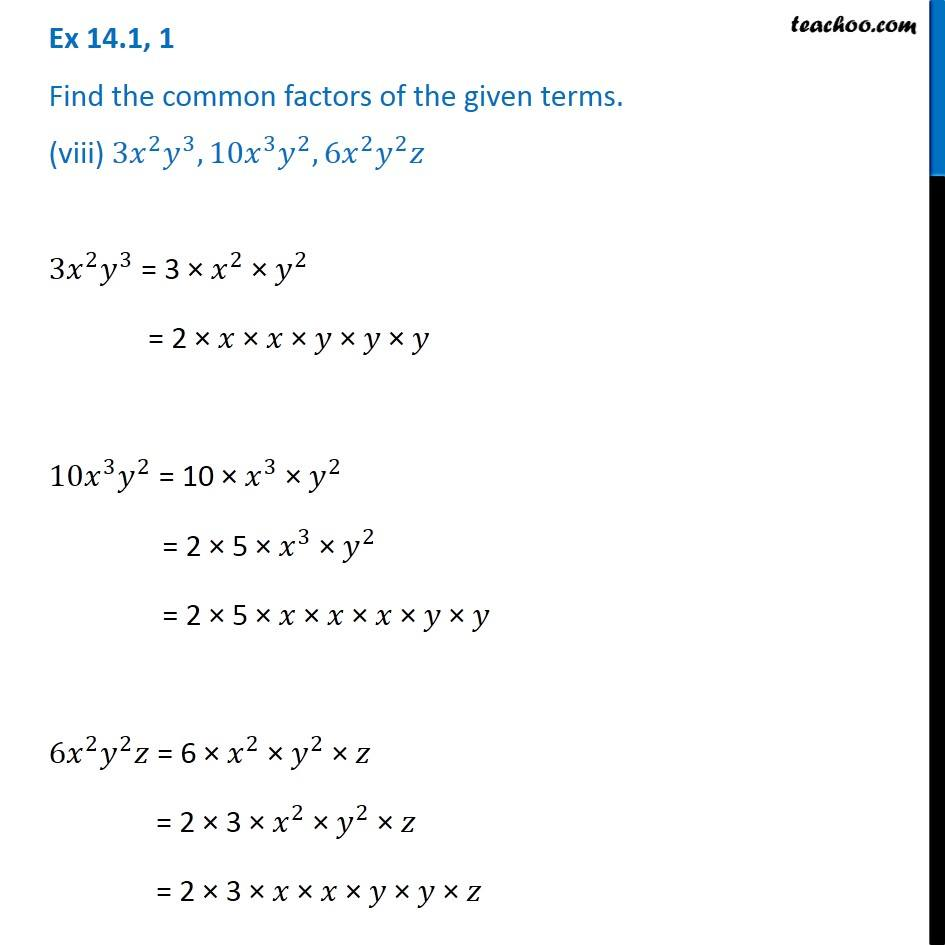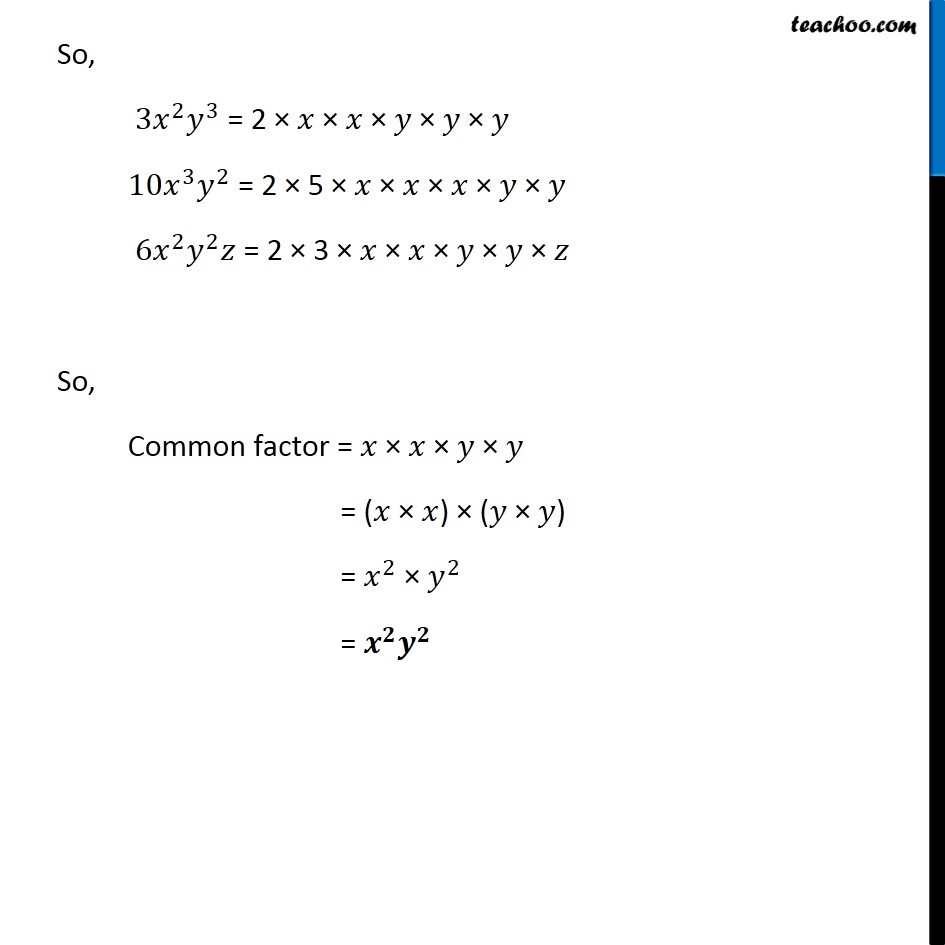Factorisation using common factors

Chapter 12 Class 8 Factorisation
Concept wiseLearn in your speed, with individual attention - Teachoo Maths 1-on-1 Class

### Transcript

Ex 12.1, 1 Find the common factors of the given terms. (viii) 〖3𝑥〗^2 𝑦^3,〖10𝑥〗^3 𝑦^2, 6𝑥^2 𝑦^2 𝑧 〖3𝑥〗^2 𝑦^3 = 3 × 𝑥^2 × 𝑦^2 = 2 × 𝑥 × 𝑥 × 𝑦 × 𝑦 × 𝑦 〖10𝑥〗^3 𝑦^2 = 10 × 𝑥^3 × 𝑦^2 = 2 × 5 × 𝑥^3 × 𝑦^2 = 2 × 5 × 𝑥 × 𝑥 × 𝑥 × 𝑦 × 𝑦 6𝑥^2 𝑦^2 𝑧 = 6 × 𝑥^2 × 𝑦^2 × 𝑧 = 2 × 3 × 𝑥^2 × 𝑦^2 × 𝑧 = 2 × 3 × 𝑥 × 𝑥 × 𝑦 × 𝑦 × 𝑧 So, 〖3𝑥〗^2 𝑦^3 = 2 × 𝑥 × 𝑥 × 𝑦 × 𝑦 × 𝑦 〖10𝑥〗^3 𝑦^2 = 2 × 5 × 𝑥 × 𝑥 × 𝑥 × 𝑦 × 𝑦 6𝑥^2 𝑦^2 𝑧 = 2 × 3 × 𝑥 × 𝑥 × 𝑦 × 𝑦 × 𝑧 So, Common factor = 𝑥 × 𝑥 × 𝑦 × 𝑦 = (𝑥 × 𝑥) × (𝑦 × 𝑦) = 𝑥^2 × 𝑦^2 = 𝒙^𝟐 𝒚^𝟐